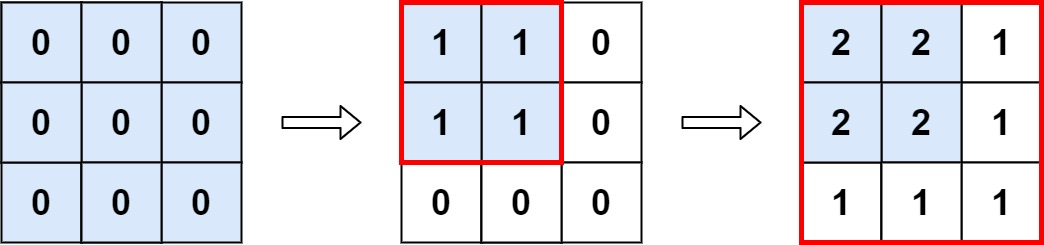## Algorithm

Problem Name: 598. Range Addition II

You are given an `m x n` matrix `M` initialized with all `0`'s and an array of operations `ops`, where `ops[i] = [ai, bi]` means `M[x][y]` should be incremented by one for all `0 <= x < ai` and `0 <= y < bi`.

Count and return the number of maximum integers in the matrix after performing all the operations.

Example 1:```Input: m = 3, n = 3, ops = [[2,2],[3,3]]
Output: 4
Explanation: The maximum integer in M is 2, and there are four of it in M. So return 4.
```

Example 2:

```Input: m = 3, n = 3, ops = [[2,2],[3,3],[3,3],[3,3],[2,2],[3,3],[3,3],[3,3],[2,2],[3,3],[3,3],[3,3]]
Output: 4
```

Example 3:

```Input: m = 3, n = 3, ops = []
Output: 9
```

Constraints:

• `1 <= m, n <= 4 * 104`
• `0 <= ops.length <= 104`
• `ops[i].length == 2`
• `1 <= ai <= m`
• `1 <= bi <= n`

## Code Examples

### #1 Code Example with Java Programming

```Code - Java Programming```

``````
public class Solution {
public int maxCount(int m, int n, int[][] ops) {
if (ops == null || ops.length == 0) {
return m * n;
}

int row = Integer.MAX_VALUE;
int col = Integer.MAX_VALUE;
for(int[] op : ops) {
row = Math.min(row, op);
col = Math.min(col, op);
}

return row * col;
}
}
``````
Copy The Code &

Input

cmd
m = 3, n = 3, ops = [[2,2],[3,3]]

Output

cmd
4

### #2 Code Example with Javascript Programming

```Code - Javascript Programming```

``````
const maxCount = function (m, n, ops) {
for (let i = 0, len = ops.length; i < len; i++) {
if (ops[i] < m) m = ops[i]
if (ops[i] < n) n = ops[i]
}
return m * n
}
``````
Copy The Code &

Input

cmd
m = 3, n = 3, ops = [[2,2],[3,3]]

Output

cmd
4

### #3 Code Example with Python Programming

```Code - Python Programming```

``````
class Solution:
def maxCount(self, m, n, ops):
"""
:type m: int
:type n: int
:type ops: List[List[int]]
:rtype: int
"""
if ops==[]:
return m*n
return min(op for op in ops)* min(op for op in ops)
``````
Copy The Code &

Input

cmd
m = 3, n = 3, ops = [[2,2],[3,3],[3,3],[3,3],[2,2],[3,3],[3,3],[3,3],[2,2],[3,3],[3,3],[3,3]]

Output

cmd
4

### #4 Code Example with C# Programming

```Code - C# Programming```

``````
using System;

namespace LeetCode
{
{
public int MaxCount(int m, int n, int[][] ops)
{
foreach (var op in ops)
{
m = Math.Min(m, op);
n = Math.Min(n, op);
}

return m * n;
}
}
}
``````
Copy The Code &

Input

cmd
m = 3, n = 3, ops = [[2,2],[3,3],[3,3],[3,3],[2,2],[3,3],[3,3],[3,3],[2,2],[3,3],[3,3],[3,3]]

Output

cmd
4